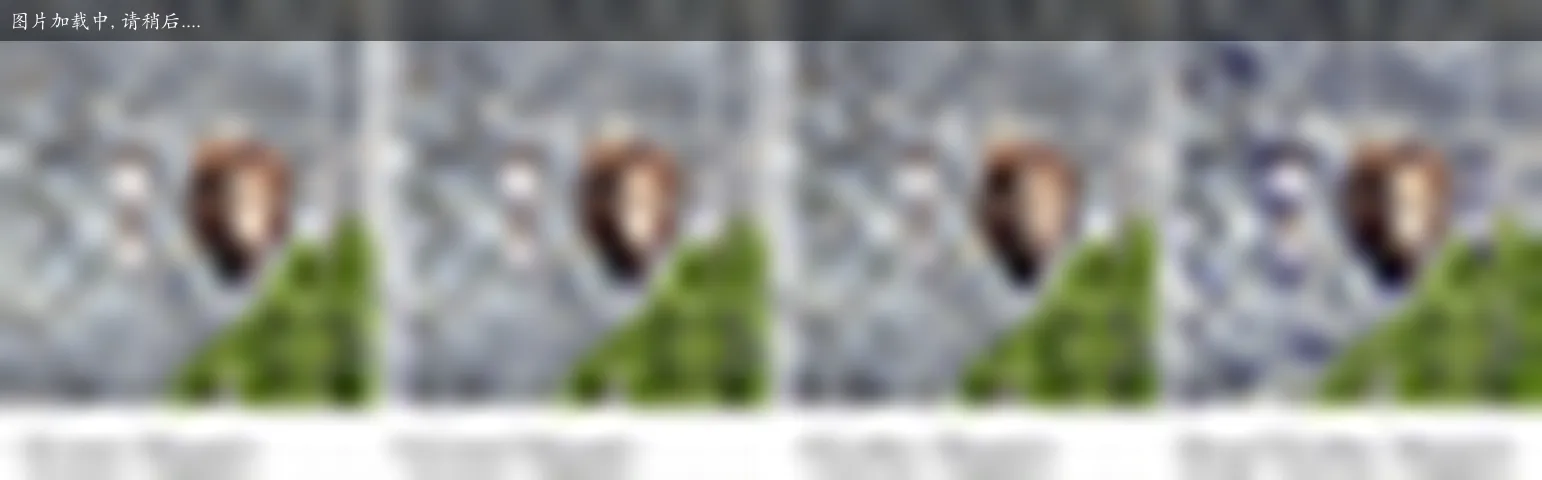Vulkan_ 法线映射、视差映射、陡视差映射和视差遮挡映射## Parallax Mapped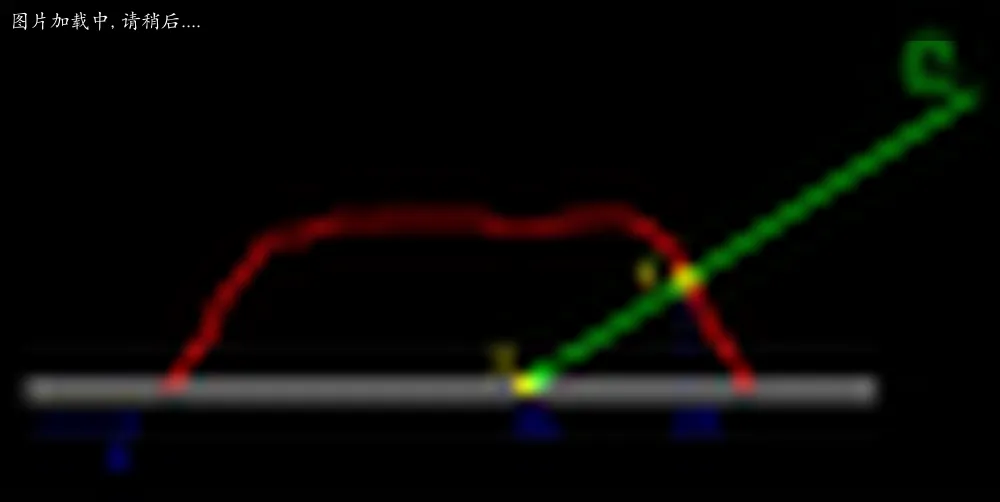// 计算 uv 的偏移 delta
inline float2 ParallaxUvDelta(v2f i)
{
// 高度图中描述的高度数据
half h = tex2D(_ParallaxMap, i.uvMain).r;
// 切线空间中的视线方向
float3 viewDir = normalize(i.viewDir);
// 将三维的视线向量投影到二维的 uv 平面，乘以高度数据
// _ParallaxScale 是一个用户可调节的值，根据效果需要进行调节，数值太大造成视觉上的严重错误
float2 delta = viewDir.xy / viewDir.z * h * _ParallaxScale;
return delta;
}

float2 uvDelta = ParallaxUvDelta(i);
i.uvMain += uvDelta;
i.uvBump += uvDelta;Parallax Mapping 的思想Parallax Mapping 的算法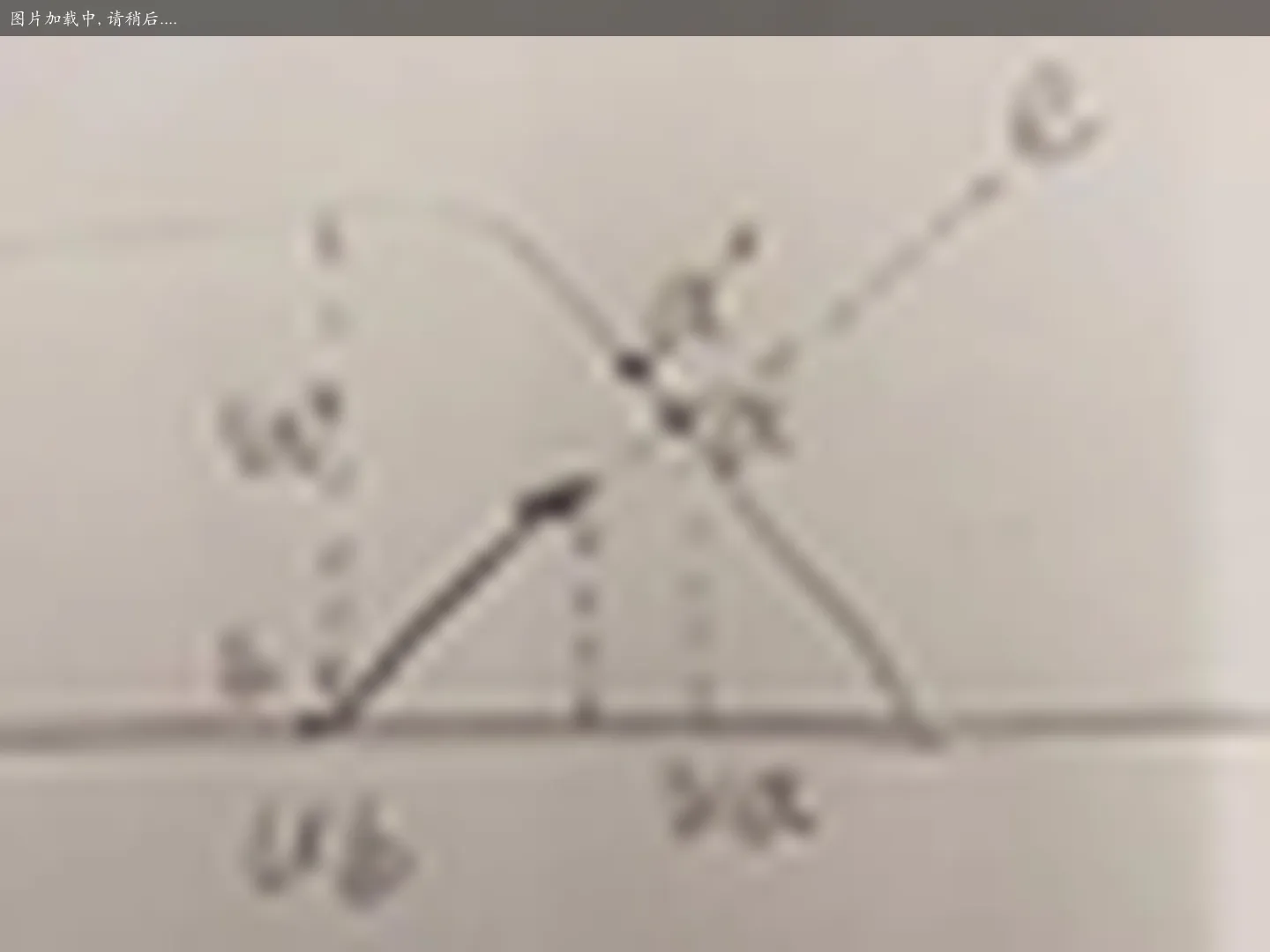uniform vec3 uEyePosition; // 相机坐标
varying vec3 vEyeVector; // 相机向量

void main()
{
vec3 EyeVector = uEyePosition - inVertexPosition;

vEyeVector.x = dot(inVertexTangent, EyeVector);
vEyeVector.y = dot(inVertexBinormal, EyeVector);
vEyeVector.z = dot(inVertexNormal, EyeVector);
vEyeVector *= vec3(1.0, -1.0, -1.0);
}

uniform float uFactor; // 纹理颜色的乘积参数
varying vec3 vEyeVector; // 相机向量

void main()
{
vec4 TempFetch = texture2D(uTextureUnit1, vTexCoord) *  2.0 - 1.0;
TempFetch *= uFactor;

vec3 EyeVector = normalize(vEyeVector);
vec2 TexCoord = EyeVector.xy * TempFetch.w + vTexCoord;

// vec4 Color  = texture2D(uTextureUnit0, vTexCoord);
vec4 Color  = texture2D(uTextureUnit0, TexCoord);
// vec3 Normal = texture2D(uTextureUnit1, vTexCoord).xyz *  2.0 - 1.0;
vec3 Normal = texture2D(uTextureUnit1, TexCoord).xyz *  2.0 - 1.0;
}


## Steep Parallax Mapped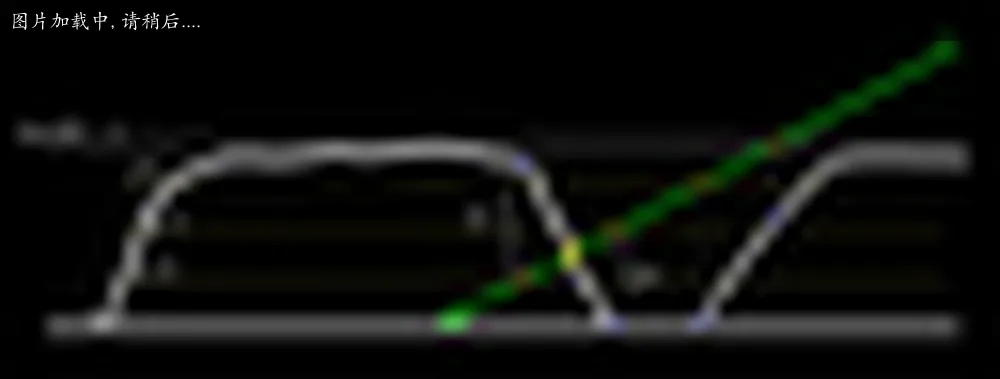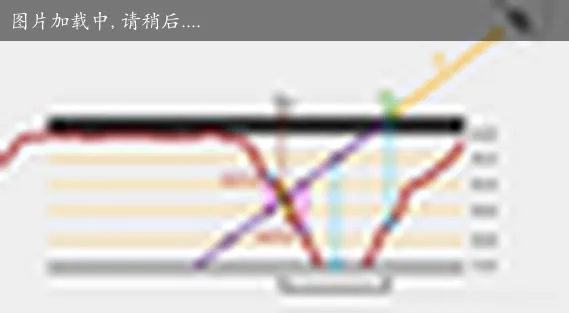inline float2 ParallaxUvDelta(Input i)
{
float3 viewDir = normalize(i.viewDir);

// 细分的层数
const float numLayers = 20;

// 单层步进的高度
float layerHeight = 1.0 / numLayers;
// 最高的高度值
float currentLayerHeight = 1.0;
// delta 最大值
float2 P = viewDir.xy * _ParallaxScale;
// delta 单步逼近值
float2 deltaTexCoords = P / numLayers;

// 开始一步步逼近，直到找到合适的红点
float2 currentTexCoords = i.uv_MainTex;
float currentDepthMapValue = tex2D(_ParallaxMap, currentTexCoords).r;
while (currentLayerHeight > currentDepthMapValue)
{
currentTexCoords -= deltaTexCoords;
currentDepthMapValue = tex2D(_ParallaxMap, currentTexCoords).r;
currentLayerHeight -= layerHeight;
}

// 计算 h1 和 h2
float2 prevTexCoords = currentTexCoords + deltaTexCoords;
float afterHeight  = currentDepthMapValue - currentLayerHeight;
float beforeHeight = currentLayerHeight + layerHeight - tex2D(_ParallaxMap, prevTexCoords).r;
// 利用 h1 h2 得到权重，在两个红点间使用权重进行差值
float weight = afterHeight / (afterHeight + beforeHeight);
float2 finalTexCoords = prevTexCoords * weight + currentTexCoords * (1.0 - weight);

return finalTexCoords - i.uv_MainTex;
}

float2 uvDelta = ParallaxUvDelta(i);
i.uvMain += uvDelta;
i.uvBump += uvDelta;


## 历史

1978 年的时候，大神 James Blinn（就是那位优化了 Phong 光照模型，将其改为 Blinn-Phong 的牛人） 在 "Simulation of Wrinkled Surfaces" 中提出了凹凸贴图（Bump mapping）。

4 年后，又有人在 Real-time relief mapping on arbitrary polygonal surfaces 一文中提出了终极加强版，浮雕贴图。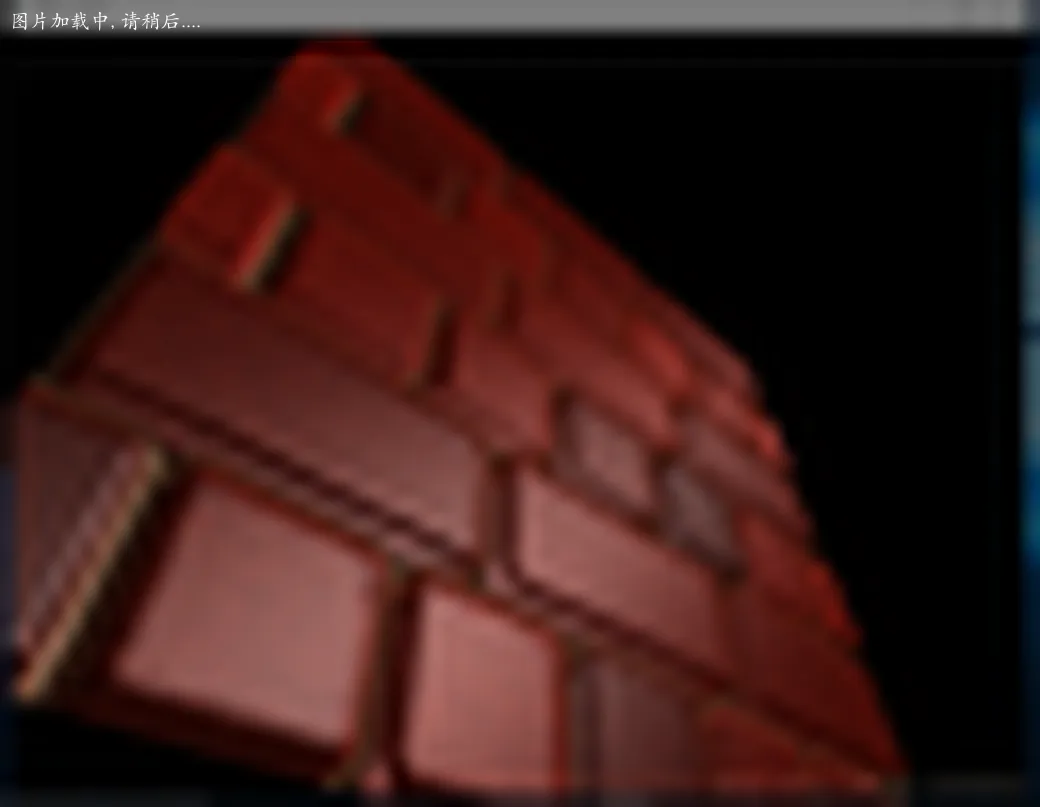parallax mapping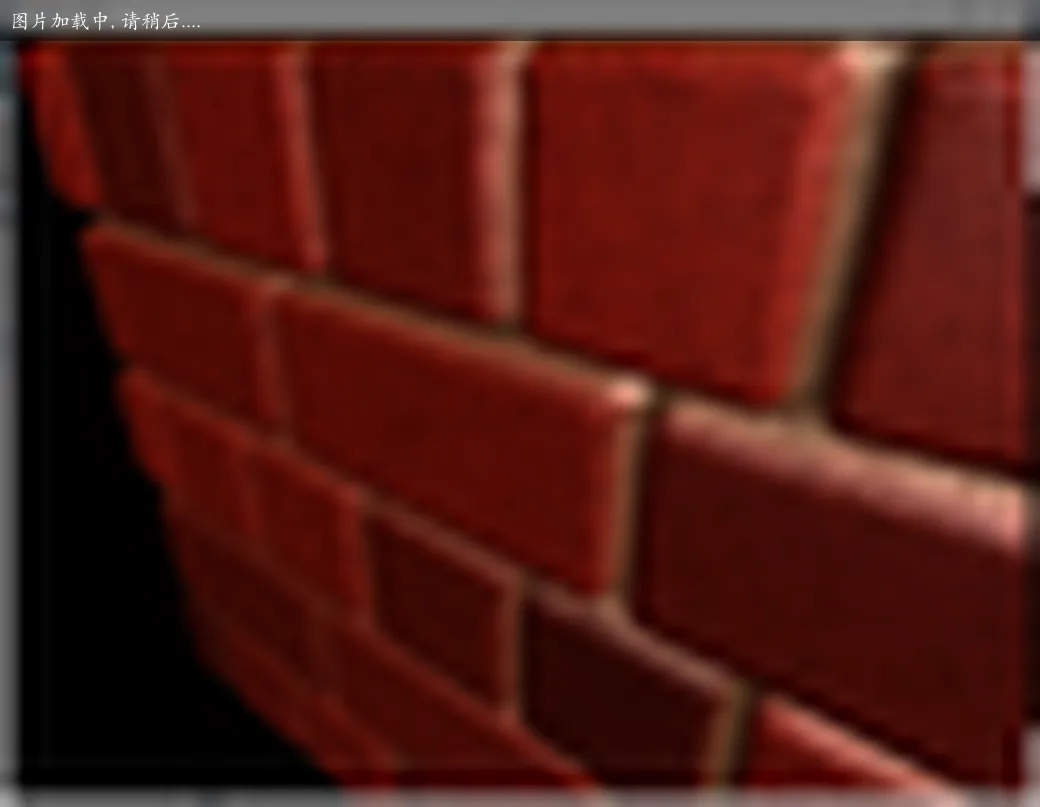steep parallax mapping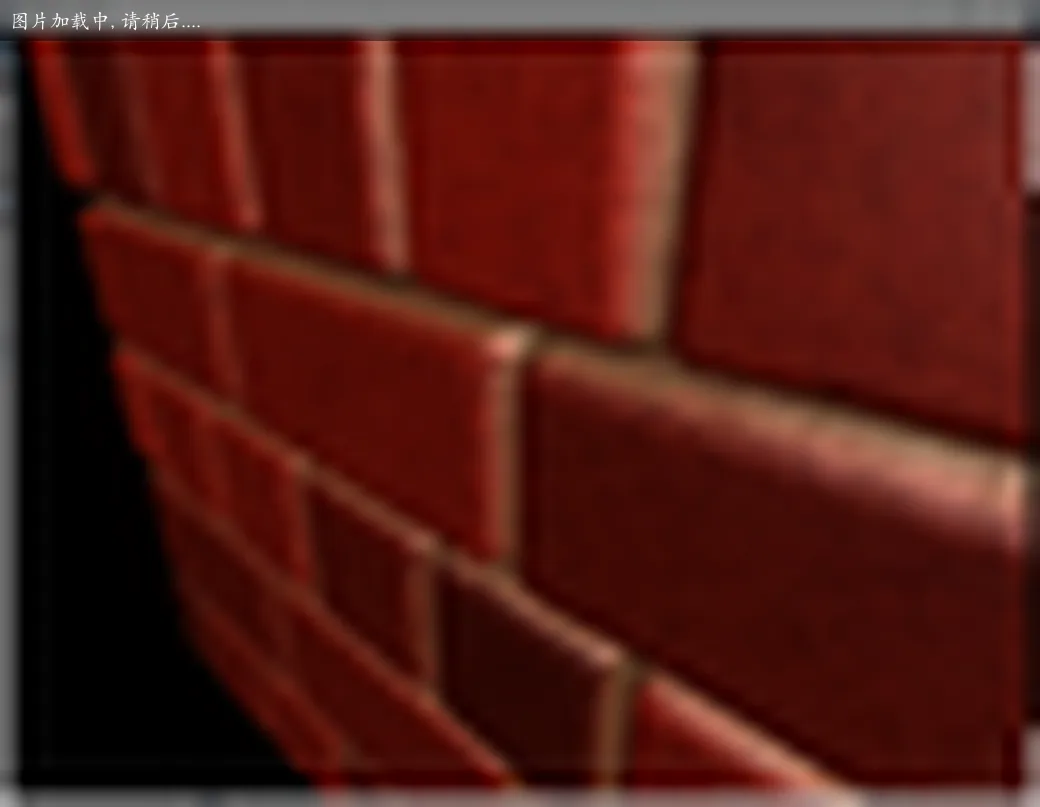steep parallax mapping 线性插值

## 需要基于物理的渲染

Physically Based Rendering。 基于物理材质是以后游戏开发的一个趋势，所以我们有必要从现在就开始逐步把制作思路从原来的模拟光照转变为现在物理真实的光照。

## Refs

LearnOpenGL 系列文章

If you have any questions or feedback, please reach out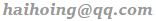.